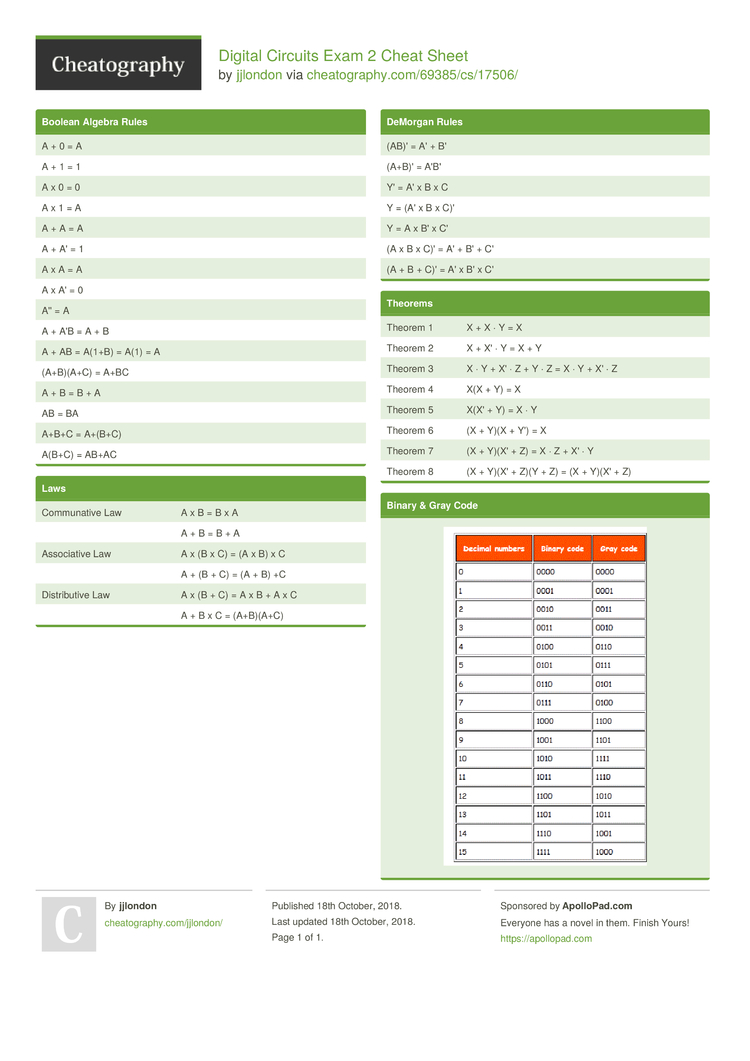# Digital Circuits Exam 2 Cheat Sheet by jjlondon

Digital Circuits

### Boolean Algebra Rules

 A + 0 = A A + 1 = 1 A x 0 = 0 A x 1 = A A + A = A A + A' = 1 A x A = A A x A' = 0 A'' = A A + A'B = A + B A + AB = A(1+B) = A(1) = A (A+B)(A+C) = A+BC A + B = B + A AB = BA A+B+C = A+(B+C) A(B+C) = AB+AC

### Laws

 Commun­­ative Law A x B = B x A A + B = B + A Associ­­ative Law A x (B x C) = (A x B) x C A + (B + C) = (A + B) +C Distri­­butive Law A x (B + C) = A x B + A x C A + B x C = (A+B)(A+C)

### DeMorgan Rules

 (AB)' = A' + B' (A+B)' = A'B' Y' = A' x B x C Y = (A' x B x C)' Y = A x B' x C' (A x B x C)' = A' + B' + C' (A + B + C)' = A' x B' x C'

### Theorems

 Theorem 1 X + X · Y = X Theorem 2 X + X' · Y = X + Y Theorem 3 X · Y + X' · Z + Y · Z = X · Y + X' · Z Theorem 4 X(X + Y) = X Theorem 5 X(X' + Y) = X · Y Theorem 6 (X + Y)(X + Y') = X Theorem 7 (X + Y)(X' + Z) = X · Z + X' · Y Theorem 8 (X + Y)(X' + Z)(Y + Z) = (X + Y)(X' + Z)

### Binary & Gray Code1 Page
//media.cheatography.com/storage/thumb/jjlondon_digital-circuits-exam-2.750.jpg

PDF (recommended)